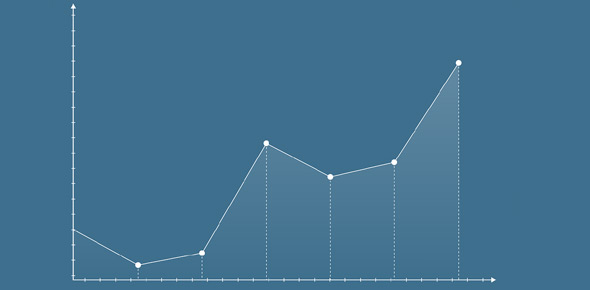# 2.3 And 2.4 Quiz

9 Questions | Total Attempts: 164Settings• 1.
Vocabulary:Which terms describe this graph? (select all that are correct)
• A.

Linear

• B.

Exponential

• C.

Discrete

• D.

Continuous

• E.

Increasing

• F.

Decreasing

• G.

Arithmetic

• H.

Geometric

• 2.
Vocabulary:Which terms describe this graph? (select all that are correct)
• A.

Linear

• B.

Exponential

• C.

Discrete

• D.

Continuous

• E.

Increasing

• F.

Decreasing

• G.

Arithmetic

• H.

Geometric

• 3.
Vocabulary:Which terms describe this graph? (select all that are correct)
• A.

Linear

• B.

Exponential

• C.

Discrete

• D.

Continuous

• E.

Increasing

• F.

Decreasing

• G.

Arithmetic

• H.

Geometric

• 4.
Vocabulary:Which terms describe this graph? (select all that are correct)
• A.

Linear

• B.

Exponential

• C.

Discrete

• D.

Continuous

• E.

Increasing

• F.

Decreasing

• G.

Arithmetic

• H.

Geometric

• 5.
Reading a Problem/Situation:Harry Potter started his first year at Hogwarts knowing 5 spells already. Each week, he learned 3 new spells. This situation begins at time [Blank]. This situation is [Blank]. It has a common difference of [Blank].
• 6.
F(n) = 5 + 3(n-0) f(15) = 50
• A.

True

• B.

False

• 7.
Which of the following equations represents this table?
• A.

F(n) = 2 - 2(n+1)

• B.

F(n) = 2 x 2^(n-1)

• C.

F(1) = 2 + 2 (n-0)

• D.

F(n) = 2 x 2^(n-0)

• 8.
Compare and contrast these graphs.Tell me at least 2 similarities (things they have in common) AND 2 differences
Related TopicsBack to top